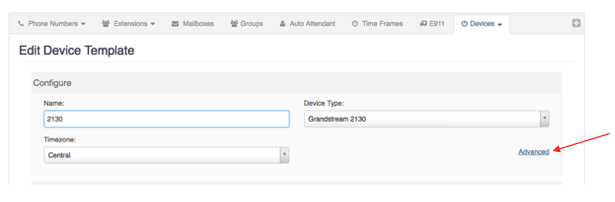# GXP2140 Sidecar Setup Guide

Note: If assistance is needed, do not hesitate to email support@cytracom.com.

To program a sidecar in the portal:

• Go to secure.cytracom.net
• Click on PBX
• Click on a Device Template or create a new oneEach key has a set of four P-values associated for programming each MPK (multi-purpose key).

1. Function - This is the set of functions that are supported by the key such as Busy Lamp Field, Monitored Call Park, or Speed Dial.
2. Account - This is the account that the function is being applied to. In some cases two extensions may be on one phone, and you need different keys for each extension registered.
3. Description - MPK display, usually a name, number or title. (In the template below, each key is labeled with the MPK#. The template can be changed as needed)
4. Value - Enter the extension, parking space, or phone number the function is going to use.

### 1. Function:

-1 - None
0 - Speed Dial
1 - BLF
2 - Presence Watcher
3 - Eventlist BLF
4 - Speed Dial via active account
5 - Dial DTMF
6 - Voice Mail
7 - Call Return
8 - Transfer
9 - Call Park
10 - Intercom
11 - LDAP Search
12 - Conference
13 - Multicast Paging
14 - Record
15 - Call Log
16 - Monitored Call Park
17 - Menu 18- XML Application
19 - Information
20 - Message

### Examples: (Setting up the first 3 MPK using the template)

(MPK 1: BLF key monitoring John Doe at Extension 101)

P23000=1
P23001=0
P23002=John Doe
P23003=101

(MPK 2: Speed dial to Jane Doe at 1234567890)

P23005=4
P23006=0
P23007=Jane Doe
P23008=123456790

(MPK 3: Parking space)

P23010=16
P23011=0
P23012=Park 1
P23013=701

### Template:

Note: The template has been pre-assembled with all keys disabled (-1) and labeled for ease of use (MPK#). It is best practice to leave all keys in the template or device but disabled for future use.

`P23000=-1P23001=0P23002=MPK1P23003=0P23005=-1P23006=0P23007=MPK2P23008=0P23010=-1P23011=0P23012=MPK3P23013=0P23015=-1P23016=0P23017=MPK4P23018=0P23020=-1P23021=0P23022=MPK5P23023=0P23025=-1P23026=0P23027=MPK6P23028=0P23030=-1P23031=0P23032=MPK7P23033=0P23035=-1P23036=0P23037=MPK8P23038=0P23040=-1P23041=0P23042=MPK9P23043=0P23045=-1P23046=0P23047=MPK10P23048=0P23050=-1P23051=0P23052=MPK11P23053=0P23055=-1P23056=0P23057=MPK12P23058=0P23060=-1P23061=0P23062=MPK13P23063=0P23065=-1P23066=0P23067=MPK14P23068=0P23070=-1P23071=0P23072=MPK15P23073=0P23075=-1P23076=0P23077=MPK16P23078=0P23080=-1P23081=0P23082=MPK17P23083=0P23085=-1P23086=0P23087=MPK18P23088=0P23090=-1P23091=0P23092=MPK19P23093=0P23095=-1P23096=0P23097=MPK20P23098=0P23100=-1P23101=0P23102=MPK21P23103=0P23105=-1P23106=0P23107=MPK22P23108=0P23110=-1P23111=0P23112=MPK23P23113=0P23115=-1P23116=0P23117=MPK24P23118=0P23120=-1P23121=0P23122=MPK25P23123=0P23125=-1P23126=0P23127=MPK26P23128=0P23130=-1P23131=0P23132=MPK27P23133=0P23135=-1P23136=0P23137=MPK28P23138=0P23140=-1P23141=0P23142=MPK29P23143=0P23145=-1P23146=0P23147=MPK30P23148=0P23150=-1P23151=0P23152=MPK31P23153=0P23155=-1P23156=0P23157=MPK32P23158=0P23160=-1P23161=0P23162=MPK33P23163=0P23165=-1P23166=0P23167=MPK34P23168=0P23170=-1P23171=0P23172=MPK35P23173=0P23175=-1P23176=0P23177=MPK36P23178=0P23180=-1P23181=0P23182=MPK37P23183=0P23185=-1P23186=0P23187=MPK38P23188=0P23190=-1P23191=0P23192=MPK39P23193=0P23195=-1P23196=0P23197=MPK40P23198=0`